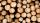# Cuprum

From 1600 mm long sheet of copper plate 2 mm thickness we have separated over the whole length the belt weighing 6000 g. Calculate belt width if 1 dm3 copper weighs 8.9 kg.

Result

b =  210.7 mm

#### Solution:Leave us a comment of example and its solution (i.e. if it is still somewhat unclear...):Be the first to comment!#### To solve this example are needed these knowledge from mathematics:

Do you know the volume and unit volume, and want to convert volume units?

## Next similar examples:

1. MilimetersThe pool is 6 meters long, 3 meters wide and the water in it is filled with water to a height 1.9 m. When John jumped into it and completely submerged, the level has risen by 4.2 mm. How much weight John when we know that one liter of the human body weighs
2. AquariumAquarium is rectangular box with square base containing 76 liters of water. Length of base edge is 42 cm. To what height the water level goes?
3. The shopThe shop has 3 hectoliters of water. How many liter bottles is it?
4. Cylindrical tank 2If a cylindrical tank with volume is used 12320cm raised to the power of 3 and base 28cm is used to store water. How many liters of water can it hold?
5. Oak cuboidOak timber is rectangular shaped with dimensions of 2m, 30 cm and 15 cm. It weight is 70 kg. Calculate the weight 1 dm³ of timber.
6. Glass doorWhat is the weight of glass door panel 5 mm thick height 2.1 meters and a width of 65 cm and 1 cubic dm of glass weighs 2.5 kg?
7. Water reservoirThe water tank has a cuboid with edges a= 1 m, b=2 m , c = 1 m. Calculate how many centimeters of water level falls, if we fill fifteen 12 liters cans.
8. HectolitersHow many hectoliters of water fits into cuboid tank with dimensions of a = 3.5 m b = 2.5 m c = 1.4 m?
9. GasolineHow many liters of gas is in the tank if its weight 15.4kg. Specific density of gasoline is 770kg/m3.
10. Two cuboidsFind the volume of cuboidal box whose one edge is: a) 1.4m and b) 2.1dm
11. Fire tankHow deep is the fire tank with the dimensions of the bottom 7m and 12m, when filled with 420 m3 of water?
12. WoodWood density is 0.6 g/cm3. How many kilograms weight 1 m3 of wood?
13. AquariumAquarium is cube with edge 45 cm. How much water can fit in there?
14. Solid in waterThe solid weighs in air 11.8 g and in water 10 g. Calculate the density of the solid.
15. Cube cornersThe wooden cube with edge 64 cm was cut in 3 corners of cube with edge 4 cm. How many cubes of edge 4 cm can be even cut?
16. Water tankWater tank shape of cuboid has dimensions of the bottom 7.5 meters and 3 meters. How high will reach the water in the tank will flow 10 liters of water per second and the inflow will be open 5/6 hour? (Calculate to one decimal place and the results give in
17. Water poolWhat water level is in the pool shaped cuboid with bottom dimensions of 25 m and 10 meters, when is 3750hl water in the pool.